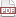### Tip 26: Safely terminated lists

22 November 11. [link]PDF version

level: follows from Tip 24 and Tip 25
purpose: operate on lists with less risk of segfaults

Part of a series of tips on POSIX and C. Start from the tip intro page, or get 21st Century C, the book based on this series.

Compound literals and variadic macros are the cutest couple, because we can now use macros to build lists and structures. I'll get to the structure building a few tips from now; let's start with lists.

Here's the sum function from a few episodes ago.

double sum(double  in[]){
double out=0;
for (int i=0; !isnan(in[i]); i++) out += in[i];
return out;
}


When using this function, you don't need to know the length of the input array, but you do need to make sure that there's a NaN marker at the end. You'll also have to check beforehand that none of your inputs are NaNs. Now the fun part, where we call this function using a variadic macro:

#include <math.h> //NAN
#include <stdio.h>

#define sum(...) sum_base((double[]){__VA_ARGS__, NAN})

double sum_base(double  in[]){
double out=0;
for (int i=0; !isnan(in[i]); i++) out += in[i];
return out;
}

int main(){
double two_and_two = sum(2, 2);
printf("2+2 = %g\n", two_and_two);
printf("(2+2)*3 = %g\n", sum(two_and_two, two_and_two, two_and_two));
printf("sum(asst) = %g\n", sum(3.1415, two_and_two, 3, 8, 98.4));
}


Now that's a stylish function. It takes in as many inputs as you have on hand, and operates on each of them. You don't have to pack the elements into an array beforehand, because the macro uses a compound initializer to do it for you.

In fact, the macro version only works with loose numbers, not with anything you've already set up as an array. If you already have an array--and if you can guarantee the NAN at the end--then you can call sum_base directly.